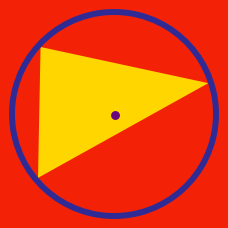Geometry

# Triangle Centers: Level 2 Challenges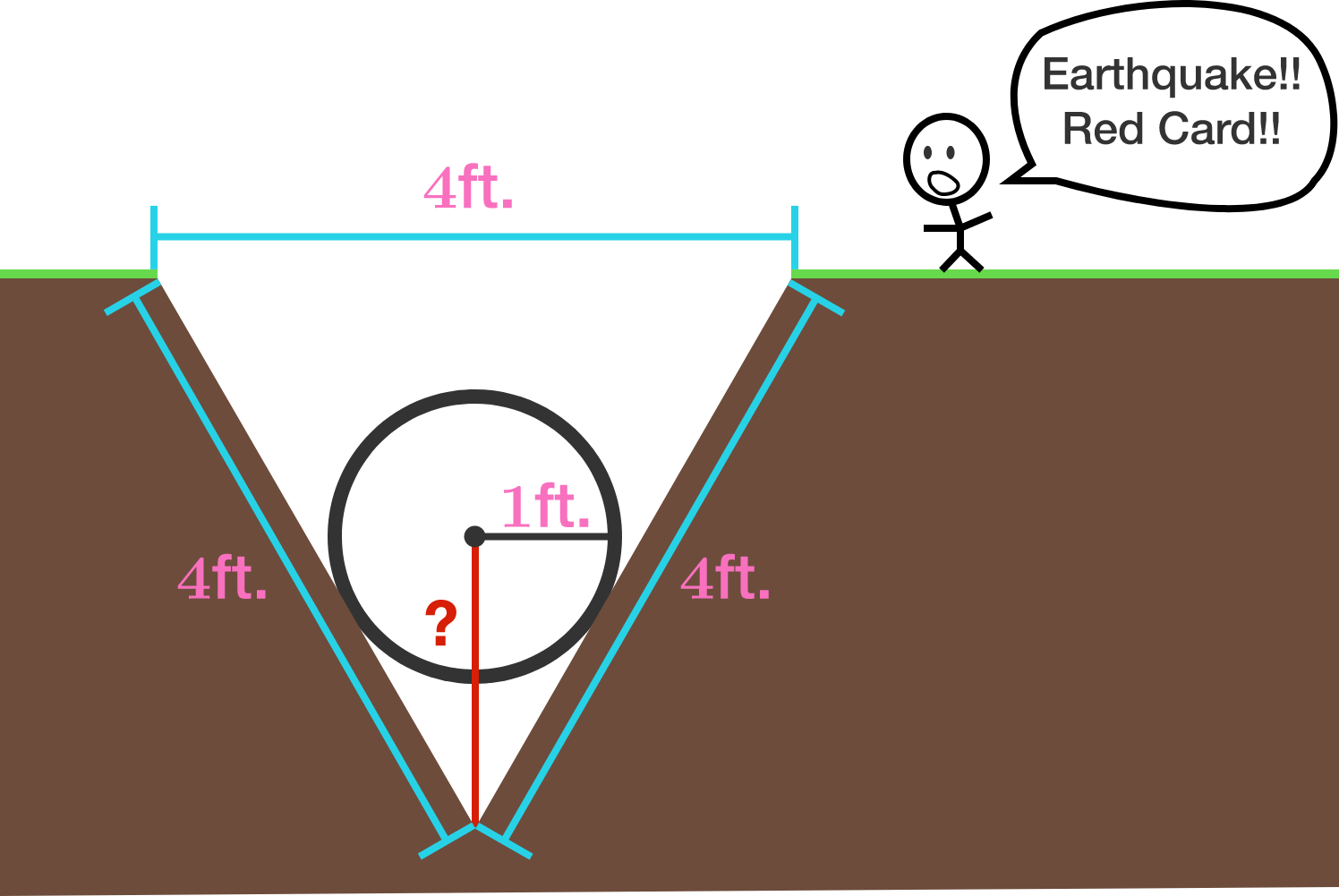At the 1920 FIFA World Cup, there was an earth quake during one of the games and a cone shaped hole was created with a diameter of 4 feet and sloping sides, both 4 feet long from ground-level to the bottom of the pit. The soccer ball, with a radius of one foot, fell into the hole. Find the distance from the center of the Soccer ball to the bottom of the pit in feet.

Assumptions:
- The sides of the hole are perfectly straight and smooth.
- The ball falls as far as it can with out changing shape.
- FYI, there wasn't actually an earthquake at the 1920 FIFA World Cup.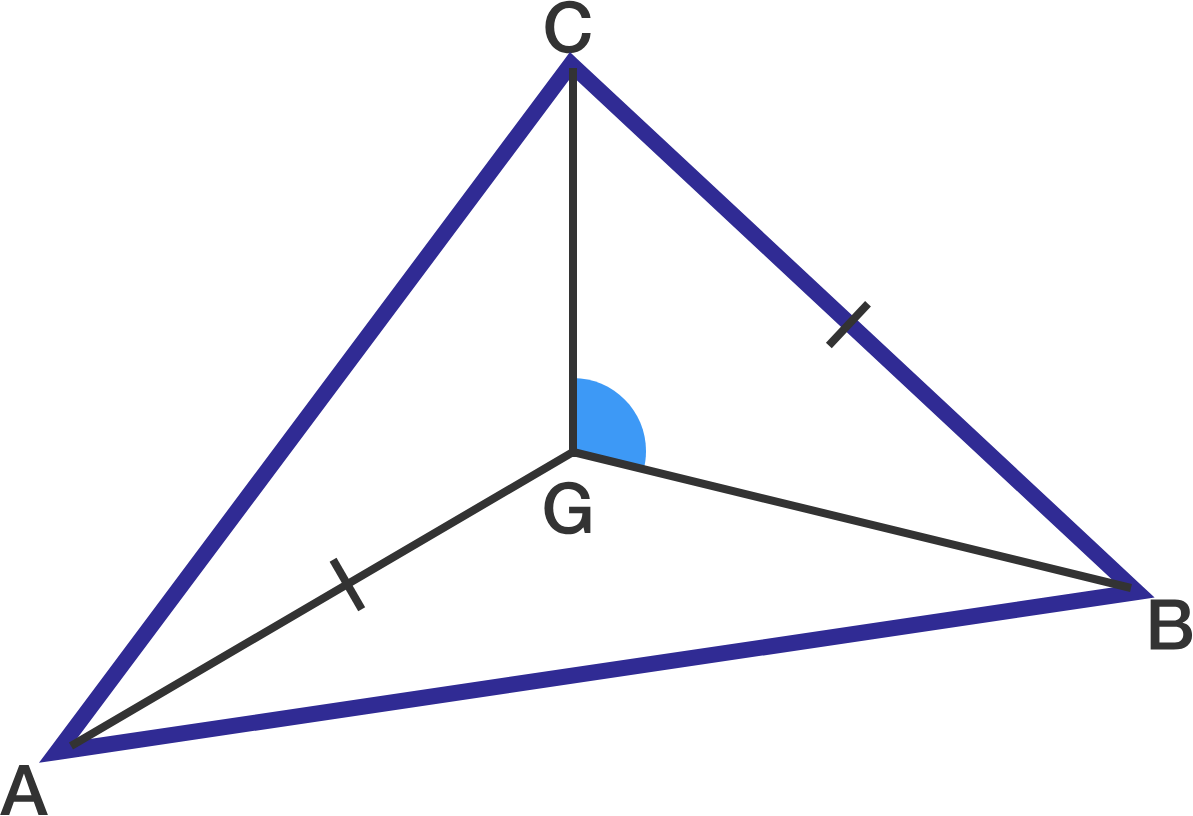In triangle $$ABC$$ with centroid $$G$$, if $$AG=BC$$, what is angle $$BGC$$ in degrees?

Note: Diagram is not drawn to scale.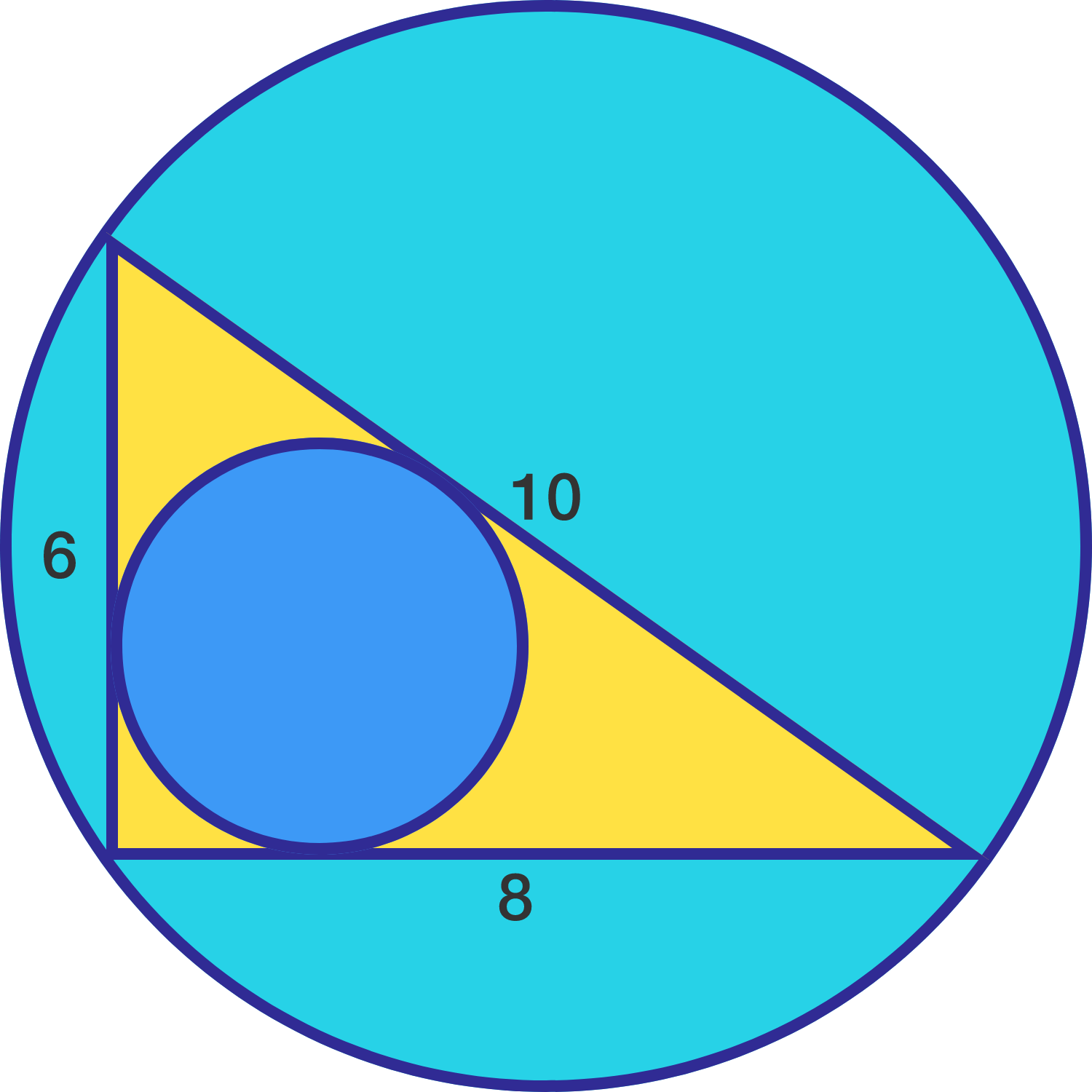A triangle has sides of 6, 8, and 10 inches.

What is the distance between incenter and circumcenter of the triangle?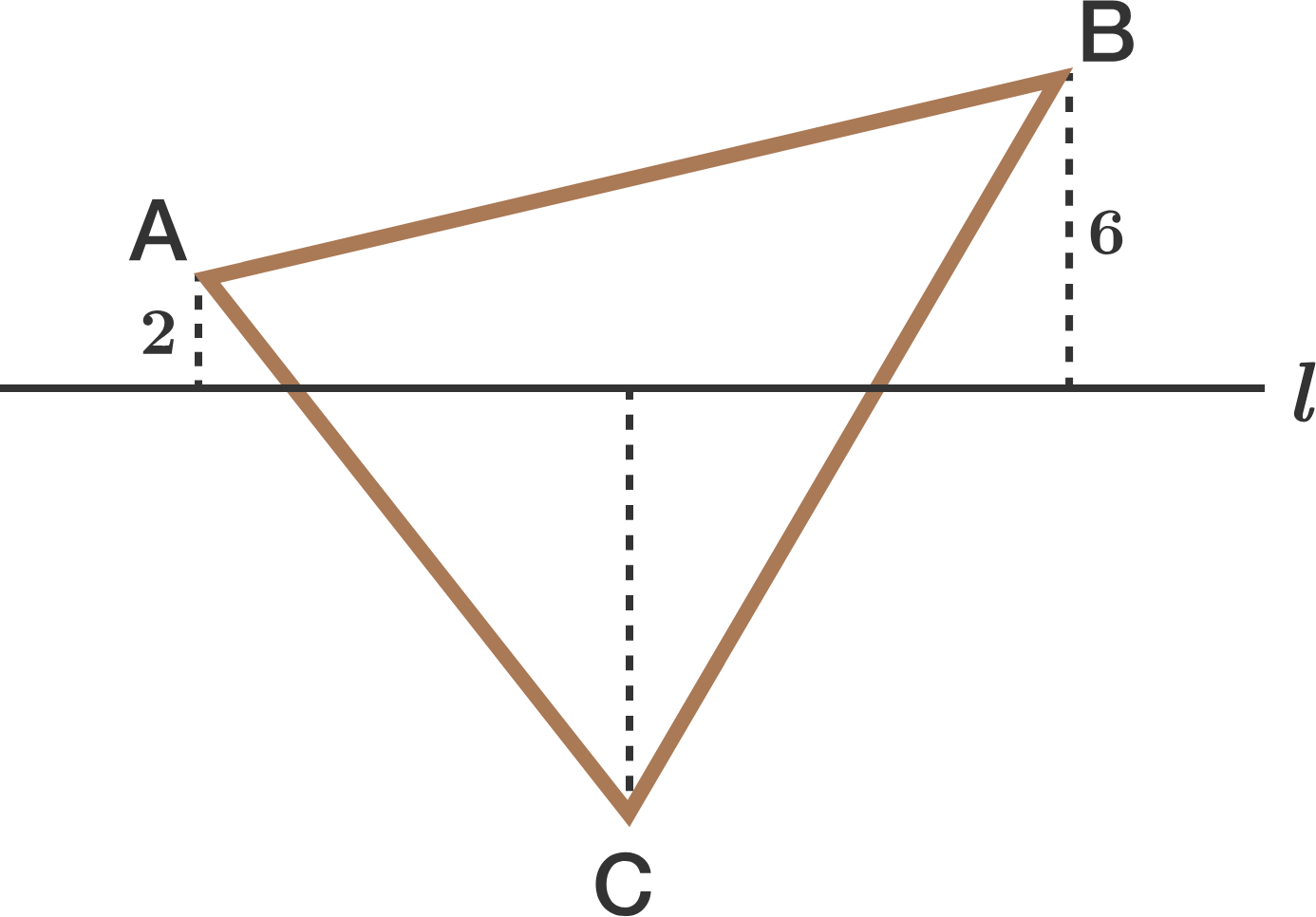In the diagram above, line $$l$$ passes through the centroid of $$\triangle ABC.$$

If the perpendicular distance between $$A$$ and line $$l$$ is 2, and the perpendicular distance between $$B$$ and line $$l$$ is 6, then what is the perpendicular distance between $$C$$ and line $$l?$$

Consider an isosceles $$\triangle ABC$$ with $$AB=AC=5, BC=6,$$ where $$I,O,H$$ denote its incenter, circumcenter, orthocenter, respectively.

Find the area of $$\triangle IOH$$.

×